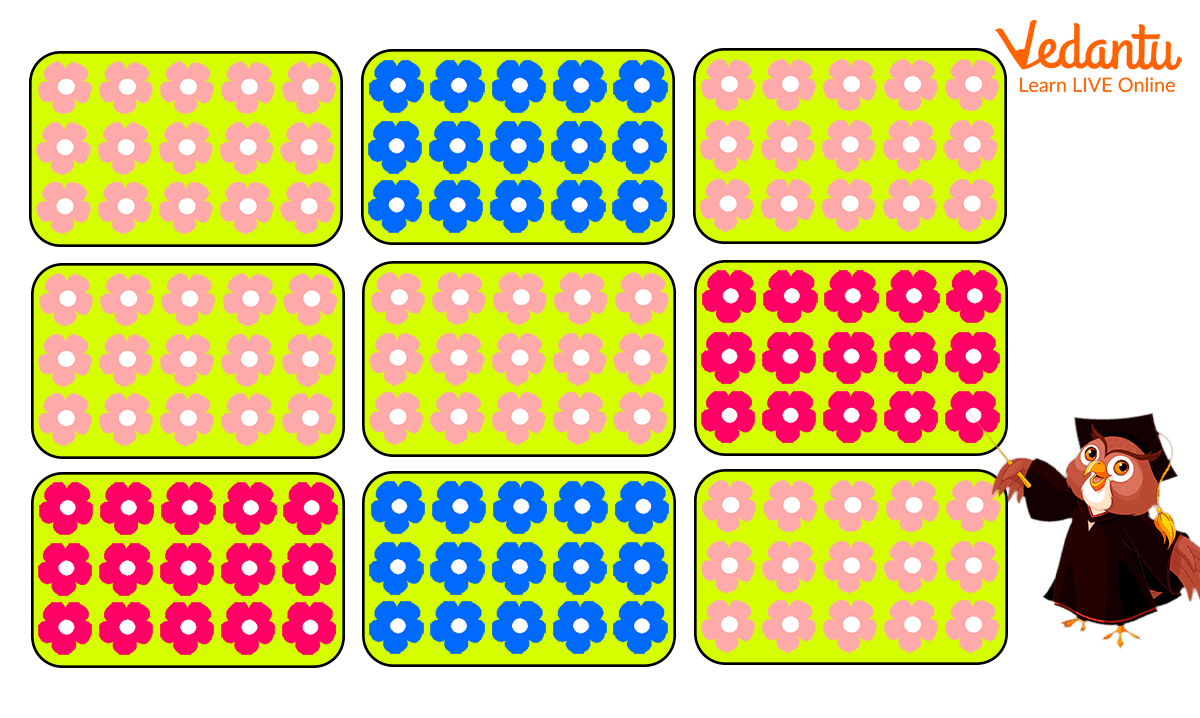Courses
Courses for Kids
Free study material
Free LIVE classes
More

# 12% as a Fraction - Converting Percentage into FractionLIVE
Join Vedantu’s FREE Mastercalss

## What is 12 as a Fraction?

What do you do when you and your sibling have to share a large pizza? To avoid a fight between you two, you divide it into several slices of the same size, isn’t it? But how do you know that each slice is of the same size? And how do you divide the pizza?

Well, here Maths comes into play. The topic of fraction in Maths defines the portion or section of a whole object or quantity. Fractions are represented in the form of a numerator and a denominator.

For example, consider this circle. The circle is divided into 12 equal parts and one part is coloured darker than the others. This darker part is called the twelfth part of the circle. In fractions, it can be written as$\frac{1}{12}$, where 1 = numerator and 12 = denominator.

Every integer is also considered a fraction with denominator 1.A circle divided into 12 equal parts.

## Convert 12% into a Fraction

12% is a term written in percentage form but we need to convert it into the fraction form. But how to do it? To express 12 as a fraction, follow the given step-by-step procedure.

Step 1: Divide the given number in percentage form by 100 like this:

12% = $\frac{12}{100}$

Step 2: Now we need to multiply both the numerator and denominator by 10 for every number after the decimal point. Since 12 is an integer with no decimal point, we can just skip to the next step.

Step 3: In this step, we need to simplify the obtained fraction, i.e. $\frac{12}{100}$ into the simplest form. To simplify any fraction, we find the greatest common divisor (GCD) of the number and then divide both the numerator and denominator by the GCD to obtain our simplified fraction. In this case, the GCD of 12 and 100 is 4.

Dividing 12/100 by 4,

(12 ÷ 4)/(100 ÷ 4) = 3/25

12 percent when written as a fraction in its simplest form is 3/25.

## Quiz Time!

1.Convert these percentages into the simplest form of fractions.

1. 18%

2. 25%

3. 76%

4. 55%

2. The given picture shows Sylvia’s garden in which she has grown flowers. Can you tell which flowers she grows the most? Express the part of those flowers as a fraction in its simplest form.Fraction exercise for kids

1. 9/50

2. 1/4

3. 19/25

4. 11/20

1. Sylivia grows red flowers the most, which are 8 in number out of a total 12 flowers.

Fraction of the field that contains red flowers = 8/12

In simplest form = 2/3

## Conclusion

Now you understand the importance of fractions and how you can express percentages as fractions. Using our detailed explanation of how to write 12 as a fraction, you can practice other similar questions yourself. Also, if you want to learn more about such mathematical concepts, explore our website and enhance your learning experience!

Last updated date: 23rd Sep 2023
Total views: 132.9k
Views today: 2.32k

## FAQs on 12% as a Fraction - Converting Percentage into Fraction

1. What are the real-life uses of fractions?

Fractions are used in almost every part of our daily lives, such as

• We read the time in terms of fractions from an analogue clock or watch.

• Splitting the restaurant bill among your friends.

• Dividing food into equal portions for the entire family.

• Comparing the prices of items in a supermarket when on sale or discounts.

2. How can you teach percentage and fractions to children?

Fraction is a fun topic to teach your young ones. You can experiment with different visual methods and hands-on activities to explain fractions to kids easily. Ask them to cut a sandwich into half or four equal parts when demonstrating the fractions$\frac{1}{2}$ or $\frac{1}{4}$. You can use slices of pizza or playdough to explain the concept of simplified fractions.